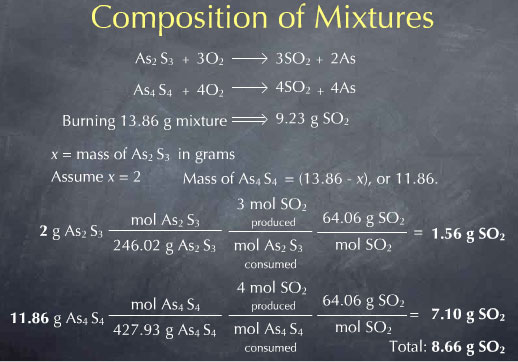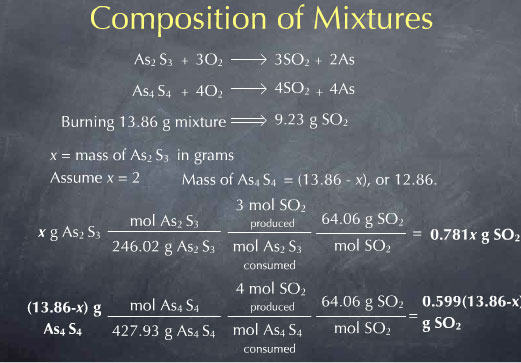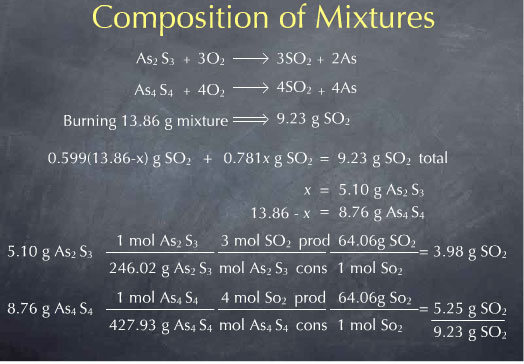# Stoichiometry Tutorials: Composition of Mixtures

(from a complete OLI stoichiometry course)

For certain mixtures, reactions can be used to determine the relative composition of a mixture. This is illustrated in the following video.

### Composition of Mixtures Movie Text

On another page, we talked about using elemental analysis as a qualitative analysis technique. In that case, we had a pure mineral and we wanted to determine what type of mineral it was. By performing an elemental analysis, we could determine the empirical formula. This can tell us, for instance, whether the mineral is As2S3 or As4S4, or something else. That approach is very useful if you think you have a pure mineral. But let's say we have a sample that we know is a mixture of two substance, such as As2S3 and As4S4. What experiment can be done to determine the relative proportion of each of these substances in our sample?

I can take a chunk of the sample and weight it. Let's say I weigh a piece of sample and find it has a mass of 13.86 grams. I then know the total mass of the mixture, but I don't know how much of it is As2S3 versus As4S4.

This is another example where chemical reactions provide a useful basis for an analytical chemistry technique. If I burn the sample, the substances react with oxygen to produce SO2 gas, leaving As metal behind. The experiment I'll perform is to burn my 13.86 grams of sample and measure the amount of SO2 gas that comes off. To see why this may be useful, consider the way that each of these minerals reacts with oxygen. The balanced reactions are shown here:

As2S3 + 3 O2 → 2 As + 3 SO2

As4S4 + 4 O2 → 4 As + 4 SO2

Since the two different minerals have different amounts of S in them, they produce different amounts of SO2. This is why measuring the amount of SO2 given off may be useful: the amount given off depends on the relative amount of the two substances in the sample. By measuring the amount of SO2 produced, I should be able to determine the amount of As2S3 versus As4S4 in the sample.

Let's say I do this experiment and my 13.86 grams of sample produces 9.23 grams of SO2. What do I do next? I don't know how many grams I have of each of these substances, I only know the total mass of the mixture and the amount of SO2 produced. This is a pretty complicated problem, and one where algebra turns out to be very useful. What algebra allows me to do is introduce a variable (let me call it x) that precisely captures what I don't know. I then write all the information I do know in terms of this unknown x. If all goes well, meaning if I have enough information from my experiment, I'll end up with an algebraic expression that I can solve for the unknown x.

The first choice I need to make here is to precisely define my unknown. Let me choose to say that x is the mass of As2S3.

x = mass of As2S3 in grams

If I knew this value, I would know everything about the sample. Before doing the algebraic solution, let's see what would happen if the sample contained 1.00g of As2S3. In other words, let me assume that the value of the unknown x is 1.00.

Since my total mass is 13.86 g, and I have 1.00 grams of As2S3, there must be 12.86 g of As4S4. I can then use reaction stoichiometry to determine the amount of SO2 produced by burning this mixture:Let's begin with the As2S3. I first use the molecular weight to convert from the number of grams of this substance to the number of molecules expressed in moles. I then look at my reaction and see that there are 3 moles of SO2 produced for every mole of As2S3 consumed. This gives me the number of SO2 molecules that are produced. To get the mass of SO2, I again use the molecular weight. This gives me the mass of SO2 produced from the 1.00 g of As2S3. A similar procedure gives me the mass of SO2 produced from the As4S4. I again use the molecular weight to convert to the moles of As4S4. From the chemical reaction, I know that 4 moles of SO2 are produced for every mole of As4S4 consumed, giving me the moles of SO2 produced. I then use the molecular weight of SO2 to convert from moles to grams. This gives me the amount of SO2 produced by burning the As4S4 portion of the sample. Experimentally, I am burning the mixture all at once, so I collect the sum of these two or 8.48 g of SO2.

This means that if my mixture contained 1.00 g of As2S3, I would expect to collect 8.48 g of SO2. In reality I collected 9.23 g of SO2. If I change the 1.00 g of As2S3 to 2.00 g, so that there is now 11.86 g of As4S4, and redo all the math, I get 8.66 g of SO2 produced.This is still not equal to observed 9.23 g of SO2 collected experimentally. I could keep guessing at the mass of As2S3 present in the sample until I get the observed 9.23 g of SO2, but it is much easier to use algebra. As mentioned above, algebra allows me define an unknown x, write everything I know in terms of this unknown, and then solve for x.

In this case, our unknown x is the mass of As2S3 in the mixture. Since the total mass is 13.86 g, the mass of As4S4 must be 13.86-x grams. Reaction stoichiometry allows me to write the amount of SO2 produced from the As2S3 as 0.781 x grams. Similarly, I get the amount of SO2 produced from the As4S4 in terms of x, as 0.599 (13.86-x) grams. I can then sum these to get the total amount of SO2 expressed in terms of my unknown x.I now now the total amount of SO2 produced by burning the mixture, in terms of the unknown x. Experimentally, I collected 9.23 g of SO2, so I can set this algebraic expression equal to 9.23

0.781 x + 0.599 (13.86-x) g SO2 total = 9.23 g SO2 total

Solving this for x gives x = 5.10. x referred to the mass of As2S3 present in the sample, so I now know that my mixture contained 5.10 grams of As2S3. The remainder of the 13.86 total mass, or 8.76 g, must then be As4S4. I now know everything I wanted to know about the sample. I can check my result by calculating the amount of SO2 produced by this mixture, and I see that I predict the observed 9.23 g of SO2.It may also be interesting to quote this result in terms of the relative proportions of the two minerals present in the mixture. As2S3 comprises 5.10 g of the 13.86 g mixture, corresponding to 36.8 %. As4S4 comprises 8.76 g of the 13.86 g mixture, or 63.2 %.I've successfully used a reaction to distinguish how much of a sample is As2S3 versus As4S4. This works because the reaction stoichiometry is different for burning As2S3 versus As4S4. Burning 1.00 gram of As2S3 produces 0.781 g of SO2, whereas burning 1.00 gram of As4S4 produces 0.599g of SO2.

Since the mass of SO2 produced on burning 1.00 g of each of these minerals is different, measuring the amount of SO2 produced on burning a mixture gives us the information we need to determine the relative amount of As2S3 and As4S4 present in the sample. This technique is a nice example of the role that reaction stoichiometry can play in quantitative analysis.

The content above has been converted from Adobe Flash Player and may not display correctly.

You may now try the following tutor on solving problems relating to mixtures.